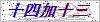# 橡胶扁平软电缆

W 型电缆具有耐气侯、抗老化和一定的耐油性能，适用于户外或接触油污的场合使用。
G 型电缆为钢丝加强型，具有一定的抗拉力作用，适用于起重机械和移动设备。
P 型电缆为屏蔽性，具有减少外界电磁波对电缆内电流的干扰，同时减少电缆内产生的电磁场对外界的影响，适用于有信号干扰的场合。

 型号 名称 主在用途 YQB、YQWB 轻型橡套扁电缆 用于轻型移动电气设备和工具 YZB、YZWB 中型橡套扁电缆 用于中型移动电气设备和工具 YCB、YCWB 重型橡套扁电缆 用于各种移动电气设备，能承受较大的机械外力的作用，特别适用于行车、起重机等电气设备。

 数×导体标称截面积mm2 电缆外形近似尺寸mm 电缆参考重量kg/km 芯数×导体标称截面积mm2 电缆外形近似尺寸mm 电缆参考重量kg/km 3×4+6×1.5 6.5×45.5 525 3×50+1×16+5×2.5+2×p1.5 17.5×76.5 2932 3×6+6×1.5 7.8×54.2 736 3×70+1×25+5×2.5+2×p1.5 19.8×86 3895 6×10+6×2.5 11×71.2 1500 3×95+1×35+5×2.5+2×p1.5 22.8×99 5124 3×10+3×6+3×4+5×2.5 19.7×68.7 2076 3×120+1×35+5×2.5+2×p1.5 25.8×112 6450 6×16+6×2.5 22.7×64.6 2378 3×120+1×35+4×4 25.8×112 6428 6×25+6×2.5 15.6×92.4 2723 3×120+2×16+4×4 28.1×99.4 6031 3×25+1×10+5×2.5+2×p1.5 14.6×63 1859 3×150+1×50+5×2.5+2×p1.5 27.6×121 7741 3×35+1×10+5×2.5+2×p1.5 14.7×63.5 2040 3×150+1×50+4×4 27.6×121 7723

：      销售专员：李

### 留言框

• #### 验证码：请输入计算结果（填写阿拉伯数字），如：三加四=70550-7532216